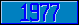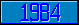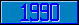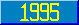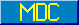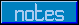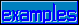## MWAPI - Position, Size and Units

### height

Introduced in the 1995 ANSI M[UMPS] Windowing Application Programmer's Interface standard.

This metalanguage symbol represents a string that starts with a numeric value, optionally followed by a comma and a specification of the unit in which the numeric value is expressed.

### hpos

Introduced in the 1995 ANSI M[UMPS] Windowing Application Programmer's Interface standard.

This metalanguage symbol represents a numeric value that indicates the horizontal location in a window. The unit of measure for this value depends on other, related, specifications.

### hscale

Introduced in the 1995 ANSI M[UMPS] Windowing Application Programmer's Interface standard.

This metalanguage symbol represents a numeric value that is used to apply horizontal relative scaling.

### hsize

Introduced in the 1995 ANSI M[UMPS] Windowing Application Programmer's Interface standard.

This metalanguage symbol represents a numeric value that specifies the horizontal dimension of an entity. The unit of measure for this value depends on other, related, specifications.

### pos

Introduced in the 1995 ANSI M[UMPS] Windowing Application Programmer's Interface standard.

This metalanguage symbol represents a position in a window. This metalanguage symbol occurs as an expression that evaluates to a string consisting of two or three pieces, separated by commas. The first piece identifies the horizontal position, the second piece identifies the vertical position. The optional third piece identifies the unit of measure.

### size

Introduced in the 1995 ANSI M[UMPS] Windowing Application Programmer's Interface standard.

This metalanguage symbol represents a specification of the dimensions of an entity. This metalanguage symbol occurs as an expression that evaluates to a string consisting of up to three pieces, separated by commas. All three pieces are optional, but at least one of the first two must be present at all times. The first piece represents the horizontal dimension. The second piece represents the vertical dimension. The third piece represents the units of measure.

### unitspec

Introduced in the 1995 ANSI M[UMPS] Windowing Application Programmer's Interface standard.

This metalanguage symbol represents a code that specifies the unit of measure for the size or position of a display, window or element. This specification can be one of a number of possibilities:

CHAR,<fontname>
PIXEL
POINT
REL,<horizontal scale>,<vertical scale>
Z<implementation-specific>

### vpos

Introduced in the 1995 ANSI M[UMPS] Windowing Application Programmer's Interface standard.

This metalanguage symbol represents a numeric value that indicates the initial vertical location in a window. The unit of measure for this value depends on other, related, specifications.

### vscale

Introduced in the 1995 ANSI M[UMPS] Windowing Application Programmer's Interface standard.

This metalanguage symbol represents a numeric value that is used to apply vertical relative scaling.

### vsize

Introduced in the 1995 ANSI M[UMPS] Windowing Application Programmer's Interface standard.

This metalanguage symbol represents a numeric value that specifies the vertical dimension of an entity. The unit of measure for this value depends on other, related, specifications.

### width

Introduced in the 1995 ANSI M[UMPS] Windowing Application Programmer's Interface standard.

This metalanguage symbol represents a string that starts with a numeric value, optionally followed by a comma and a specification of the unit in which the numeric value is expressed.